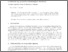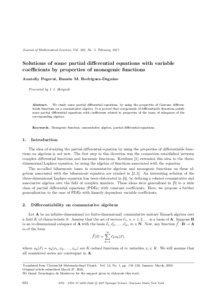# Solutions of some partial differential equations with variable coefficients by properties of monogenic functions

Pogoruі, А. А. and Rodríguez-Dagnіno, Ramón М. (2017) Solutions of some partial differential equations with variable coefficients by properties of monogenic functions. Journal of Mathematical Sciences, 220 (5). pp. 624-632.Preview
TextView Item